# Software Analytics In Action: A Hands-on Tutorial on Mining, Analyzing, Modelling, and Explaining Software Data

26 de May de 2019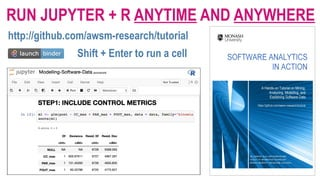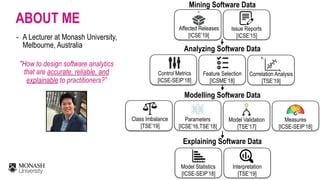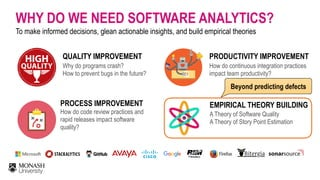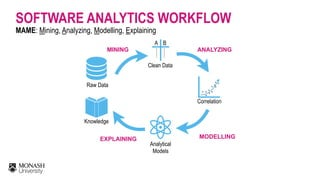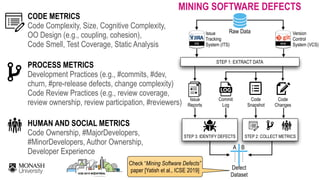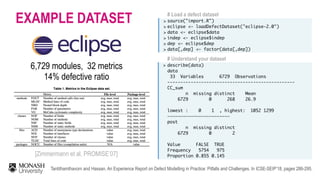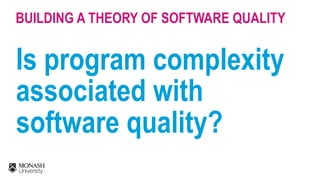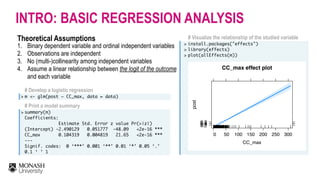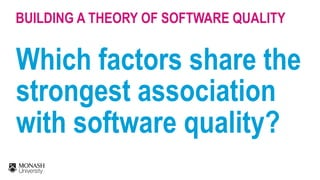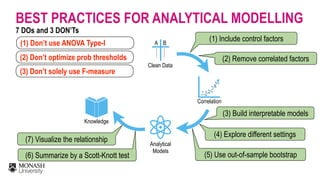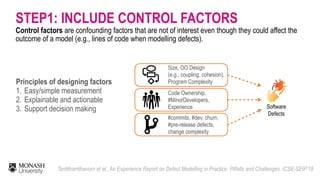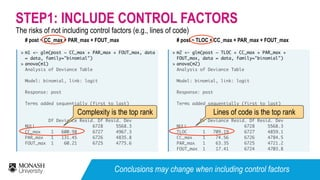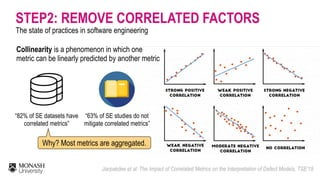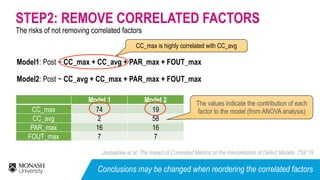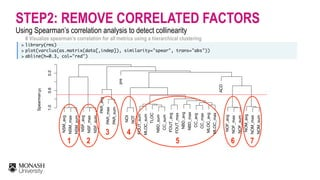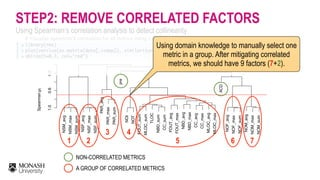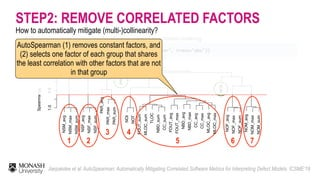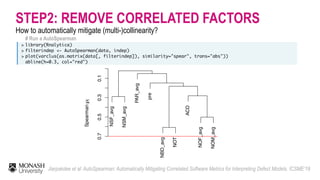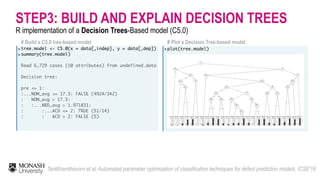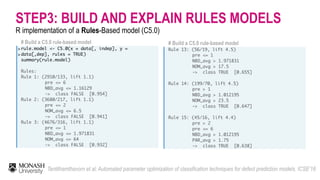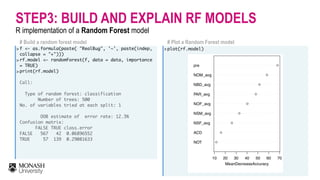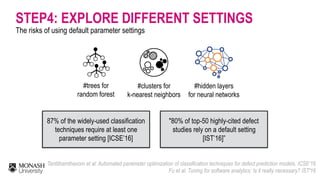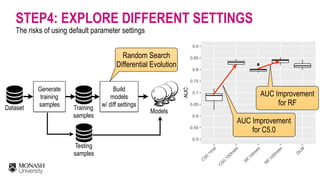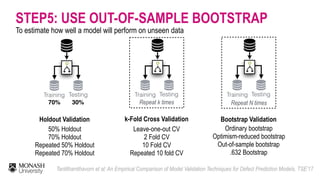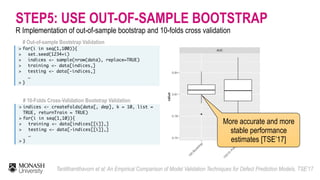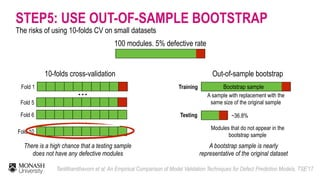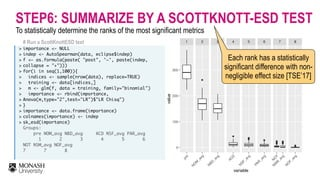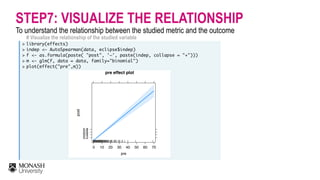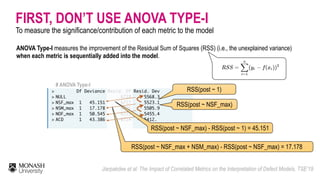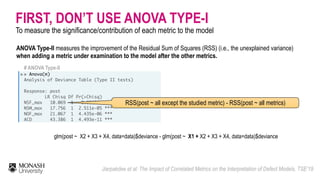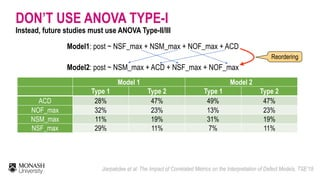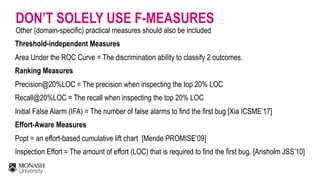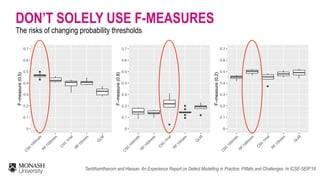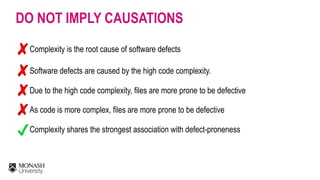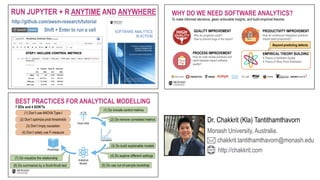1 de 36

### Software Analytics In Action: A Hands-on Tutorial on Mining, Analyzing, Modelling, and Explaining Software Data

• 1. A Hands-on Tutorial on Analyzing and Modelling Software Data Dr. Chakkrit (Kla) Tantithamthavorn Software Analytics in Action Monash University, Australia. chakkrit.tantithamthavorn@monash.edu @klainfohttp://chakkrit.com
• 2. RUN JUPYTER + R ANYTIME AND ANYWHERE http://github.com/awsm-research/tutorial Shift + Enter to run a cell
• 3. Mining Software Data Analyzing Software Data Affected Releases  [ICSE’19] Issue Reports  [ICSE’15] Control Metrics  [ICSE-SEIP’18] Feature Selection  [ICSME’18] Correlation Analysis  [TSE’19] Modelling Software Data Class Imbalance  [TSE’19] Parameters  [ICSE’16,TSE’18] Model Validation  [TSE’17] Measures  [ICSE-SEIP’18] Explaining Software Data Model Statistics  [ICSE-SEIP’18] Interpretation  [TSE’19] ABOUT ME - A Lecturer at Monash University, Melbourne, Australia "How to design software analytics that are accurate, reliable, and explainable to practitioners?”
• 4. WHY DO WE NEED SOFTWARE ANALYTICS? To make informed decisions, glean actionable insights, and build empirical theories PROCESS IMPROVEMENT How do code review practices and rapid releases impact software quality? PRODUCTIVITY IMPROVEMENT How do continuous integration practices impact team productivity? QUALITY IMPROVEMENT Why do programs crash? How to prevent bugs in the future? EMPIRICAL THEORY BUILDING A Theory of Software Quality A Theory of Story Point Estimation Beyond predicting defects
• 5. SOFTWARE ANALYTICS WORKFLOW MAME: Mining, Analyzing, Modelling, Explaining Raw Data …… …… A B Clean Data MINING Correlation . . . .. . . . . ..   ANALYZING Analytical   Models   MODELLING Knowledge   EXPLAINING
• 6. ITS VCS Issue   Tracking  System (ITS) Version  Control  System (VCS) Raw Data Code Changes Code Snapshot Commit Log Issue   Reports STEP 1: EXTRACT DATA STEP 3: IDENTIFY DEFECTS STEP 2: COLLECT METRICS …… …… A B Defect  Dataset CODE METRICS Code Complexity, Size, Cognitive Complexity,  OO Design (e.g., coupling, cohesion),   Code Smell, Test Coverage, Static Analysis PROCESS METRICS Development Practices (e.g., #commits, #dev,   churn, #pre-release defects, change complexity)   Code Review Practices (e.g., review coverage,  review ownership, review participation, #reviewers) HUMAN AND SOCIAL METRICS Code Ownership, #MajorDevelopers,   #MinorDevelopers, Author Ownership,  Developer Experience MINING SOFTWARE DEFECTS Check “Mining Software Defects” paper [Yatish et al., ICSE 2019]
• 7. EXAMPLE DATASET # Load a defect dataset > > > > >  > source("import.R")  eclipse <- loadDefectDataset("eclipse-2.0") data <- eclipse\$data indep <- eclipse\$indep dep <- eclipse\$dep data[,dep] <- factor(data[,dep]) 6,729 modules, 32 metrics  14% defective ratio Tantithamthavorn and Hassan. An Experience Report on Defect Modelling in Practice: Pitfalls and Challenges. In ICSE-SEIP’18, pages 286-295. # Understand your dataset > describe(data) data 33 Variables 6729 Observations ------------------------------------------------- CC_sum n missing distinct Mean 6729 0 268 26.9 lowest : 0 1 , highest: 1052 1299 ———————————————————————— post n missing distinct 6729 0 2 Value FALSE TRUE Frequency 5754 975 Proportion 0.855 0.145[Zimmermann et al, PROMISE’07]
• 8. Is program complexity associated with software quality? BUILDING A THEORY OF SOFTWARE QUALITY
• 9. # Develop a logistic regression > m <- glm(post ~ CC_max, data = data) # Print a model summary > summary(m) Coefficients: Estimate Std. Error z value Pr(>|z|) (Intercept) -2.490129 0.051777 -48.09 <2e-16 *** CC_max 0.104319 0.004819 21.65 <2e-16 ***  --- Signif. codes: 0 ‘***’ 0.001 ‘**’ 0.01 ‘*’ 0.05 ‘.’ 0.1 ‘ ’ 1 INTRO: BASIC REGRESSION ANALYSIS Theoretical Assumptions 1. Binary dependent variable and ordinal independent variables 2. Observations are independent 3. No (multi-)collinearity among independent variables 4. Assume a linear relationship between the logit of the outcome and each variable # Visualize the relationship of the studied variable > > > install.packages("effects") library(effects) plot(allEffects(m))                                  CC_max effect plot CC_max post 0.20.40.60.8 0 50 100 150 200 250 300
• 10. Which factors share the strongest association with software quality? BUILDING A THEORY OF SOFTWARE QUALITY
• 11. …… …… A B Knowledge Analytical   Models Clean Data Correlation . . . .. . . . . .. BEST PRACTICES FOR ANALYTICAL MODELLING (1) Include control factors (3) Build interpretable models (4) Explore different settings (2) Remove correlated factors (7) Visualize the relationship (5) Use out-of-sample bootstrap(6) Summarize by a Scott-Knott test (1) Don’t use ANOVA Type-I (2) Don’t optimize prob thresholds 7 DOs and 3 DON’Ts (3) Don’t solely use F-measure
• 12. STEP1: INCLUDE CONTROL FACTORS Size, OO Design   (e.g., coupling, cohesion), Program Complexity Software Defects Control factors are confounding factors that are not of interest even though they could affect the outcome of a model (e.g., lines of code when modelling defects). #commits, #dev, churn,   #pre-release defects,   change complexity Code Ownership,  #MinorDevelopers, Experience Principles of designing factors 1. Easy/simple measurement 2. Explainable and actionable 3. Support decision making Tantithamthavorn et al., An Experience Report on Defect Modelling in Practice: Pitfalls and Challenges. ICSE-SEIP’18
• 13. The risks of not including control factors (e.g., lines of code) STEP1: INCLUDE CONTROL FACTORS # post ~ CC_max + PAR_max + FOUT_max  > > m1 <- glm(post ~ CC_max + PAR_max + FOUT_max, data = data, family="binomial") anova(m1) Analysis of Deviance Table Model: binomial, link: logit Response: post Terms added sequentially (first to last) Df Deviance Resid. Df Resid. Dev NULL 6728 5568.3 CC_max 1 600.98 6727 4967.3 PAR_max 1 131.45 6726 4835.8 FOUT_max 1 60.21 6725 4775.6  # post ~ TLOC + CC_max + PAR_max + FOUT_max  > > m2 <- glm(post ~ TLOC + CC_max + PAR_max + FOUT_max, data = data, family="binomial") anova(m2)  Analysis of Deviance Table Model: binomial, link: logit Response: post Terms added sequentially (first to last) Df Deviance Resid. Df Resid. Dev NULL 6728 5568.3 TLOC 1 709.19 6727 4859.1 CC_max 1 74.56 6726 4784.5 PAR_max 1 63.35 6725 4721.2 FOUT_max 1 17.41 6724 4703.8 Complexity is the top rank Lines of code is the top rank Conclusions may change when including control factors
• 14. STEP2: REMOVE CORRELATED FACTORS The state of practices in software engineering Jiarpakdee et al: The Impact of Correlated Metrics on the Interpretation of Defect Models. TSE’19 “82% of SE datasets have   correlated metrics” “63% of SE studies do not   mitigate correlated metrics” Why? Most metrics are aggregated. Collinearity is a phenomenon in which one metric can be linearly predicted by another metric
• 15. The risks of not removing correlated factors STEP2: REMOVE CORRELATED FACTORS Model 1 Model 2 CC_max 74 19 CC_avg 2 58 PAR_max 16 16 FOUT_max 7 7 Model1: Post ~ CC_max + CC_avg + PAR_max + FOUT_max Model2: Post ~ CC_avg + CC_max + PAR_max + FOUT_max CC_max is highly correlated with CC_avg The values indicate the contribution of each factor to the model (from ANOVA analysis) Jiarpakdee et al: The Impact of Correlated Metrics on the Interpretation of Defect Models. TSE’19 Conclusions may be changed when reordering the correlated factors
• 16. # Visualize spearman’s correlation for all metrics using a hierarchical clustering > > > library(rms) plot(varclus(as.matrix(data[,indep]), similarity="spear", trans="abs")) abline(h=0.3, col="red") STEP2: REMOVE CORRELATED FACTORS Using Spearman’s correlation analysis to detect collinearity 1 NSM_avg NSM_max NSM_sum NSF_avg NSF_max NSF_sum PAR_avg PAR_max PAR_sum pre NOI NOT FOUT_sum MLOC_sum TLOC NBD_sum CC_sum FOUT_avg FOUT_max NBD_avg NBD_max CC_avg CC_max MLOC_avg MLOC_max ACD NOF_avg NOF_max NOF_sum NOM_avg NOM_max NOM_sum 1.00.60.2 Spearmanρ 2 4 5 3 6 7
• 17. # Visualize spearman’s correlation for all metrics using a hierarchical clustering > > > library(rms) plot(varclus(as.matrix(data[,indep]), similarity="spear", trans="abs")) abline(h=0.3, col="red") STEP2: REMOVE CORRELATED FACTORS Using Spearman’s correlation analysis to detect collinearity 1 NSM_avg NSM_max NSM_sum NSF_avg NSF_max NSF_sum PAR_avg PAR_max PAR_sum pre NOI NOT FOUT_sum MLOC_sum TLOC NBD_sum CC_sum FOUT_avg FOUT_max NBD_avg NBD_max CC_avg CC_max MLOC_avg MLOC_max ACD NOF_avg NOF_max NOF_sum NOM_avg NOM_max NOM_sum 1.00.60.2 Spearmanρ 2 4 Using domain knowledge to manually select one metric in a group. After mitigating correlated metrics, we should have 9 factors (7+2). A GROUP OF CORRELATED METRICS NON-CORRELATED METRICS 5 3 6 7
• 18. # Visualize spearman’s correlation for all metrics using a hierarchical clustering > > > library(rms) plot(varclus(as.matrix(data[,indep]), similarity="spear", trans="abs")) abline(h=0.3, col="red") STEP2: REMOVE CORRELATED FACTORS 1 NSM_avg NSM_max NSM_sum NSF_avg NSF_max NSF_sum PAR_avg PAR_max PAR_sum pre NOI NOT FOUT_sum MLOC_sum TLOC NBD_sum CC_sum FOUT_avg FOUT_max NBD_avg NBD_max CC_avg CC_max MLOC_avg MLOC_max ACD NOF_avg NOF_max NOF_sum NOM_avg NOM_max NOM_sum 1.00.60.2 Spearmanρ 2 3 4 5 6 AutoSpearman (1) removes constant factors, and (2) selects one factor of each group that shares the least correlation with other factors that are not in that group 7 How to automatically mitigate (multi-)collinearity? Jiarpakdee et al: AutoSpearman: Automatically Mitigating Correlated Software Metrics for Interpreting Defect Models. ICSME’18
• 19. # Run a AutoSpearman > > > library(Rnalytica) filterindep <- AutoSpearman(data, indep) plot(varclus(as.matrix(data[, filterindep]), similarity="spear", trans="abs")) abline(h=0.3, col="red") STEP2: REMOVE CORRELATED FACTORS How to automatically mitigate (multi-)collinearity? NSF_avg NSM_avg PAR_avg pre NBD_avg NOT ACD NOF_avg NOM_avg 0.70.50.30.1 Spearmanρ Jiarpakdee et al: AutoSpearman: Automatically Mitigating Correlated Software Metrics for Interpreting Defect Models. ICSME’18
• 20. STEP3: BUILD AND EXPLAIN DECISION TREES R implementation of a Decision Trees-Based model (C5.0) # Build a C5.0 tree-based model > > tree.model <- C5.0(x = data[,indep], y = data[,dep]) summary(tree.model) Read 6,729 cases (10 attributes) from undefined.data Decision tree: pre <= 1: :...NOM_avg <= 17.5: FALSE (4924/342) : NOM_avg > 17.5: : :...NBD_avg > 1.971831: : :...ACD <= 2: TRUE (51/14) : : ACD > 2: FALSE (5)    Tantithamthavorn et al: Automated parameter optimization of classification techniques for defect prediction models. ICSE’16 # Plot a Decision Tree-based model >plot(tree.model)                            pre 1 ≤ 1 > 1 NOM_avg 2 ≤ 17.5 > 17.5 Node 3 (n = 4924) TRUEFALSE 0 0.2 0.4 0.6 0.8 1 NBD_avg 4 ≤ 1.972 > 1.972 NBD_avg 5 ≤ 1.029 > 1.029 Node 6 (n = 64) TRUEFALSE 0 0.2 0.4 0.6 0.8 1 NOM_avg 7 ≤ 64 > 64 Node 8 (n = 332) TRUEFALSE 0 0.2 0.4 0.6 0.8 1 Node 9 (n = 21) TRUEFALSE 0 0.2 0.4 0.6 0.8 1 ACD 10 ≤ 2 > 2 Node 11 (n = 51) TRUEFALSE 0 0.2 0.4 0.6 0.8 1 Node 12 (n = 5) TRUEFALSE 0 0.2 0.4 0.6 0.8 1 pre 13 ≤ 6 > 6 NBD_avg 14 ≤ 1.012 > 1.012 Node 15 (n = 180) TRUEFALSE 0 0.2 0.4 0.6 0.8 1 NOM_avg 16 ≤ 23.5 > 23.5 PAR_avg 17 ≤ 0.677 > 0.677 PAR_avg 18 ≤ 0.579 > 0.579 NBD_avg 19 ≤ 2.833> 2.833 Node 20 (n = 118) TRUEFALSE 0 0.2 0.4 0.6 0.8 1 Node 21 (n = 7) TRUEFALSE 0 0.2 0.4 0.6 0.8 1 NBD_avg 22 ≤ 1.564> 1.564 Node 23 (n = 70) TRUEFALSE 0 0.2 0.4 0.6 0.8 1 Node 24 (n = 29) TRUEFALSE 0 0.2 0.4 0.6 0.8 1 pre 25 ≤ 2 > 2 NBD_avg 26 ≤ 2.13 > 2.13 Node 27 (n = 188) TRUEFALSE 0 0.2 0.4 0.6 0.8 1 NOF_avg 28 ≤ 1.75 > 1.75 Node 29 (n = 29) TRUEFALSE 0 0.2 0.4 0.6 0.8 1 NOM_avg 30 ≤ 6.5 > 6.5 Node 31 (n = 10) TRUEFALSE 0 0.2 0.4 0.6 0.8 1 Node 32 (n = 15) TRUEFALSE 0 0.2 0.4 0.6 0.8 1 PAR_avg 33 ≤ 1.75 > 1.75 Node 34 (n = 288) TRUEFALSE 0 0.2 0.4 0.6 0.8 1 NBD_avg 35 ≤ 1.161> 1.161 Node 36 (n = 4) TRUEFALSE 0 0.2 0.4 0.6 0.8 1 Node 37 (n = 37) TRUEFALSE 0 0.2 0.4 0.6 0.8 1 ACD 38 ≤ 0 > 0 NSF_avg 39 ≤ 11 > 11 Node 40 (n = 73) TRUEFALSE 0 0.2 0.4 0.6 0.8 1 Node 41 (n = 12) TRUEFALSE 0 0.2 0.4 0.6 0.8 1 NOF_avg 42 ≤ 12 > 12 Node 43 (n = 27) TRUEFALSE 0 0.2 0.4 0.6 0.8 1 Node 44 (n = 7) TRUEFALSE 0 0.2 0.4 0.6 0.8 1 pre 45 ≤ 12 > 12 NSM_avg 46 ≤ 0.25 > 0.25 NOF_avg 47 ≤ 10.5 > 10.5 Node 48 (n = 94) TRUEFALSE 0 0.2 0.4 0.6 0.8 1 Node 49 (n = 11) TRUEFALSE 0 0.2 0.4 0.6 0.8 1 Node 50 (n = 56) TRUEFALSE 0 0.2 0.4 0.6 0.8 1 Node 51 (n = 77) TRUEFALSE 0 0.2 0.4 0.6 0.8 1
• 21. STEP3: BUILD AND EXPLAIN RULES MODELS R implementation of a Rules-Based model (C5.0) Tantithamthavorn et al: Automated parameter optimization of classification techniques for defect prediction models. ICSE’16 # Build a C5.0 rule-based model Rule 13: (56/19, lift 4.5) pre <= 1 NBD_avg > 1.971831 NOM_avg > 17.5 -> class TRUE [0.655] Rule 14: (199/70, lift 4.5) pre > 1 NBD_avg > 1.012195 NOM_avg > 23.5 -> class TRUE [0.647] Rule 15: (45/16, lift 4.4) pre > 2 pre <= 6 NBD_avg > 1.012195 PAR_avg > 1.75 -> class TRUE [0.638] # Build a C5.0 rule-based model > > rule.model <- C5.0(x = data[, indep], y = data[,dep], rules = TRUE) summary(rule.model)    Rules: Rule 1: (2910/133, lift 1.1) pre <= 6 NBD_avg <= 1.16129 -> class FALSE [0.954] Rule 2: (3680/217, lift 1.1) pre <= 2 NOM_avg <= 6.5 -> class FALSE [0.941]  Rule 3: (4676/316, lift 1.1) pre <= 1 NBD_avg <= 1.971831 NOM_avg <= 64 -> class FALSE [0.932]
• 22. STEP3: BUILD AND EXPLAIN RF MODELS R implementation of a Random Forest model # Build a random forest model > > > f <- as.formula(paste( "RealBug", '~', paste(indep, collapse = "+"))) rf.model <- randomForest(f, data = data, importance = TRUE) print(rf.model)    Call: Type of random forest: classification Number of trees: 500 No. of variables tried at each split: 1 OOB estimate of error rate: 12.3% Confusion matrix: FALSE TRUE class.error FALSE 567 42 0.06896552 TRUE 57 139 0.29081633      # Plot a Random Forest model >plot(rf.model)                                            NOT ACD NSF_avg NSM_avg NOF_avg PAR_avg NBD_avg NOM_avg pre ● ● ● ● ● ● ● ● ● 10 20 30 40 50 60 70 MeanDecreaseAccuracy NOT ACD NSM_avg NSF_avg NOF_avg pre NOM_avg PAR_avg NBD_avg ● 0 rf.model
• 23. STEP4: EXPLORE DIFFERENT SETTINGS The risks of using default parameter settings Tantithamthavorn et al: Automated parameter optimization of classification techniques for defect prediction models. ICSE’16  Fu et al. Tuning for software analytics: Is it really necessary? IST'16 87% of the widely-used classification techniques require at least one parameter setting [ICSE’16] #trees for   random forest #clusters for   k-nearest neighbors #hidden layers for neural networks "80% of top-50 highly-cited defect studies rely on a default setting [IST’16]”
• 24. STEP4: EXPLORE DIFFERENT SETTINGS The risks of using default parameter settings Dataset Generate training samples Training  samples Testing  samples Models Build   models  w/ diff settings Random Search  Differential Evolution ●● 0.5 0.55 0.6 0.65 0.7 0.75 0.8 0.85 0.9 C 50.1trial C 50.100trials R F.10trees R F.100trees G LM AUC AUC Improvement  for C5.0 AUC Improvement  for RF
• 25. STEP5: USE OUT-OF-SAMPLE BOOTSTRAP To estimate how well a model will perform on unseen data Tantithamthavorn et al: An Empirical Comparison of Model Validation Techniques for Defect Prediction Models. TSE’17 Testing 70% 30% Training Holdout Validation k-Fold Cross Validation Repeat k times Bootstrap Validation 50% Holdout 70% Holdout Repeated 50% Holdout Repeated 70% Holdout Leave-one-out CV 2 Fold CV 10 Fold CV Repeated 10 fold CV Ordinary bootstrap Optimism-reduced bootstrap Out-of-sample bootstrap .632 Bootstrap TestingTraining Repeat N times TestingTraining
• 26. STEP5: USE OUT-OF-SAMPLE BOOTSTRAP R Implementation of out-of-sample bootstrap and 10-folds cross validation Tantithamthavorn et al: An Empirical Comparison of Model Validation Techniques for Defect Prediction Models. TSE’17 # Out-of-sample Bootstrap Validation > > > > > > for(i in seq(1,100)){ set.seed(1234+i) indices <- sample(nrow(data), replace=TRUE) training <- data[indices,] testing <- data[-indices,] … } # 10-Folds Cross-Validation Bootstrap Validation > > > > > indices <- createFolds(data[, dep], k = 10, list = TRUE, returnTrain = TRUE) for(i in seq(1,10)){ training <- data[indices[[i]],] testing <- data[-indices[[i]],] … } ● ● AUC 100 Bootstrap 10X10−Fold C V 0.75 0.78 0.81 0.84 value More accurate and more stable performance estimates [TSE’17]
• 27. Fold 1 100 modules, 5% defective rate 10-folds cross-validation Fold 5 Fold 6 … Fold 10 There is a high chance that a testing sample does not have any defective modules … Out-of-sample bootstrap Training Testing A sample with replacement with the same size of the original sample Modules that do not appear in the bootstrap sample Bootstrap sample ~36.8% A bootstrap sample is nearly representative of the original dataset STEP5: USE OUT-OF-SAMPLE BOOTSTRAP The risks of using 10-folds CV on small datasets Tantithamthavorn et al: An Empirical Comparison of Model Validation Techniques for Defect Prediction Models. TSE’17
• 28. STEP6: SUMMARIZE BY A SCOTTKNOTT-ESD TEST To statistically determine the ranks of the most significant metrics # Run a ScottKnottESD test > > > > > > > > > > > > > > importance <- NULL indep <- AutoSpearman(data, eclipse\$indep) f <- as.formula(paste( "post", '~', paste(indep, collapse = "+"))) for(i in seq(1,100)){ indices <- sample(nrow(data), replace=TRUE) training <- data[indices,] m <- glm(f, data = training, family="binomial") importance <- rbind(importance, Anova(m,type="2",test="LR")\$"LR Chisq") } importance <- data.frame(importance) colnames(importance) <- indep sk_esd(importance) Groups: pre NOM_avg NBD_avg ACD NSF_avg PAR_avg 1 2 3 4 5 6   NOT NSM_avg NOF_avg  7 7 8 ● ● ●● ● ● ● ●● ● ● ●● ● ● ●●●●● ● ●●● ● ●●●●●●●● ● 1 2 3 4 5 6 7 8 pre N O M _avg N BD _avg AC D N SF_avg PAR _avg N O T N SM _avg N O F_avg 0 100 200 300 variablevalue Each rank has a statistically significant difference with non- negligible effect size [TSE’17]
• 29. # Visualize the relationship of the studied variable > > > > > library(effects) indep <- AutoSpearman(data, eclipse\$indep) f <- as.formula(paste( "post", '~', paste(indep, collapse = "+"))) m <- glm(f, data = data, family="binomial") plot(effect("pre",m))                                STEP7: VISUALIZE THE RELATIONSHIP To understand the relationship between the studied metric and the outcome pre effect plot pre post 0.2 0.4 0.6 0.8 0 10 20 30 40 50 60 70
• 30. # ANOVA Type-I > > > > > > Df Deviance Resid. Df Resid. Dev NULL 6728 5568.3 NSF_max 1 45.151 6727 5523.1 NSM_max 1 17.178 6726 5505.9 NOF_max 1 50.545 6725 5455.4 ACD 1 43.386 6724 5412. FIRST, DON’T USE ANOVA TYPE-I To measure the significance/contribution of each metric to the model Jiarpakdee et al: The Impact of Correlated Metrics on the Interpretation of Defect Models. TSE’19 RSS(post ~ 1) RSS(post ~ NSF_max) ANOVA Type-I measures the improvement of the Residual Sum of Squares (RSS) (i.e., the unexplained variance) when each metric is sequentially added into the model. RSS(post ~ NSF_max) - RSS(post ~ 1) = 45.151 RSS(post ~ NSF_max + NSM_max) - RSS(post ~ NSF_max) = 17.178
• 31. FIRST, DON’T USE ANOVA TYPE-I To measure the significance/contribution of each metric to the model Jiarpakdee et al: The Impact of Correlated Metrics on the Interpretation of Defect Models. TSE’19 # ANOVA Type-II > > Anova(m) Analysis of Deviance Table (Type II tests) Response: post LR Chisq Df Pr(>Chisq) NSF_max 10.069 1 0.001508 ** NSM_max 17.756 1 2.511e-05 *** NOF_max 21.067 1 4.435e-06 *** ACD 43.386 1 4.493e-11 *** RSS(post ~ all except the studied metric) - RSS(post ~ all metrics) ANOVA Type-II measures the improvement of the Residual Sum of Squares (RSS) (i.e., the unexplained variance) when adding a metric under examination to the model after the other metrics. glm(post ~ X2 + X3 + X4, data=data)\$deviance - glm(post ~ X1 + X2 + X3 + X4, data=data)\$deviance
• 32. DON’T USE ANOVA TYPE-I Instead, future studies must use ANOVA Type-II/III Jiarpakdee et al: The Impact of Correlated Metrics on the Interpretation of Defect Models. TSE’19 Model 1 Model 2 Type 1 Type 2 Type 1 Type 2 ACD 28% 47% 49% 47% NOF_max 32% 23% 13% 23% NSM_max 11% 19% 31% 19% NSF_max 29% 11% 7% 11% Model1: post ~ NSF_max + NSM_max + NOF_max + ACD Model2: post ~ NSM_max + ACD + NSF_max + NOF_max Reordering
• 33. DON’T SOLELY USE F-MEASURES Other (domain-specific) practical measures should also be included Threshold-independent Measures  Area Under the ROC Curve = The discrimination ability to classify 2 outcomes. Ranking Measures  Precision@20%LOC = The precision when inspecting the top 20% LOC  Recall@20%LOC = The recall when inspecting the top 20% LOC  Initial False Alarm (IFA) = The number of false alarms to find the first bug [Xia ICSME’17]  Effort-Aware Measures  Popt = an effort-based cumulative lift chart [Mende PROMISE’09]  Inspection Effort = The amount of effort (LOC) that is required to find the first bug. [Arisholm JSS’10]
• 34. DON’T SOLELY USE F-MEASURES ● ● 0 0.1 0.2 0.3 0.4 0.5 0.6 0.7 C 50.100trials R F.100trees C 50.1trial R F.10trees G LM F−measure(0.5) ● ● ● ● ● ● 0 0.1 0.2 0.3 0.4 0.5 0.6 0.7 C 50.100trials R F.100trees C 50.1trial R F.10trees G LM F−measure(0.8) ● 0 0.1 0.2 0.3 0.4 0.5 0.6 0.7 C 50.100trials R F.100trees C 50.1trial R F.10trees G LM F−measure(0.2) Tantithamthavorn and Hassan. An Experience Report on Defect Modelling in Practice: Pitfalls and Challenges. In ICSE-SEIP’18 The risks of changing probability thresholds
• 35. DO NOT IMPLY CAUSATIONS Complexity is the root cause of software defects Software defects are caused by the high code complexity. Due to the high code complexity, files are more prone to be defective As code is more complex, files are more prone to be defective Complexity shares the strongest association with defect-proneness
• 36. Dr. Chakkrit (Kla) Tantithamthavorn Monash University, Australia. chakkrit.tantithamthavorn@monash.edu http://chakkrit.com